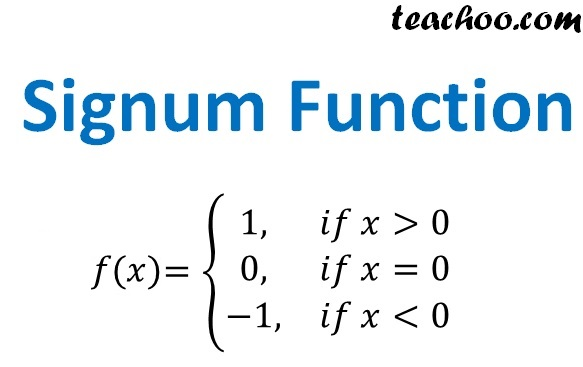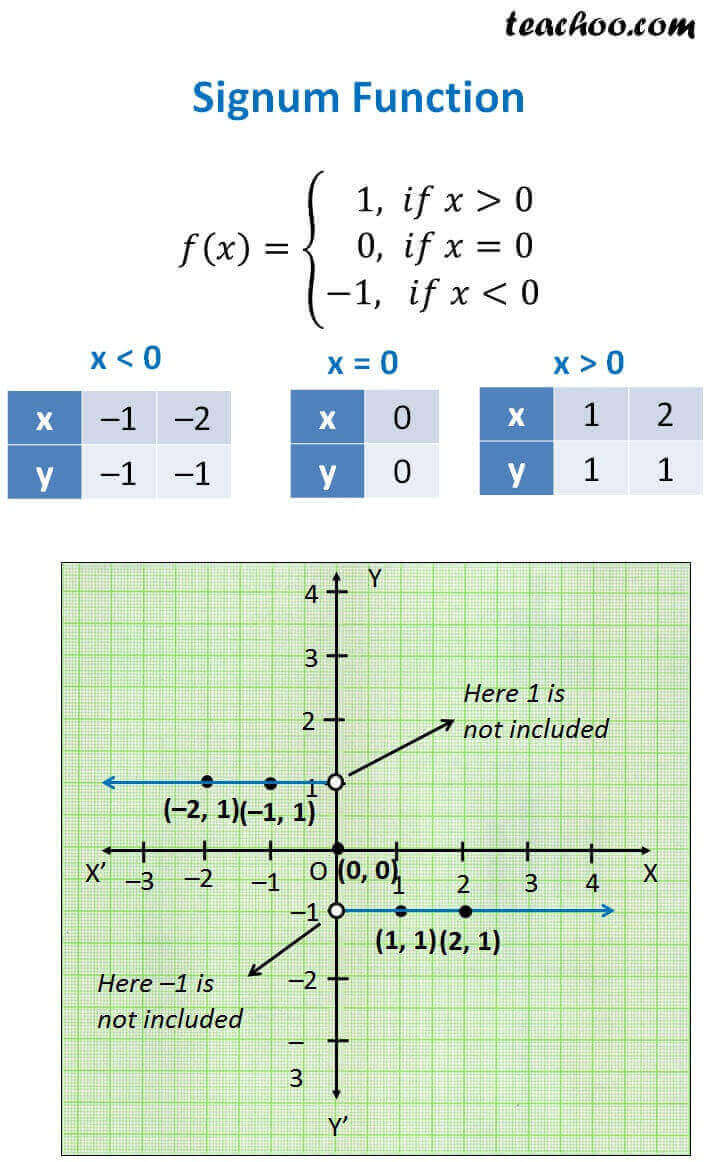Different Functions and their graphs

Chapter 2 Class 11 Relations and Functions
Concept wise

f: R RThis is known as signum function.

Let us check value of f(x) for different values of x

x < 0

So, f(x) = –1

x < 0

So, f(x) = –1

x > 0

So, f(x) = 1

x > 0

So, f(x) = 1

#### For x = 0

x = 0

So, f(x) = 0

Now,

Plotting graphHere,

Domain = All values of x = R

Range = All values of y

Since y will have value 0, 1 or –1

Range = {0, 1, –1}

Learn in your speed, with individual attention - Teachoo Maths 1-on-1 Class